## Chebyshev Polynomial of the Second Kind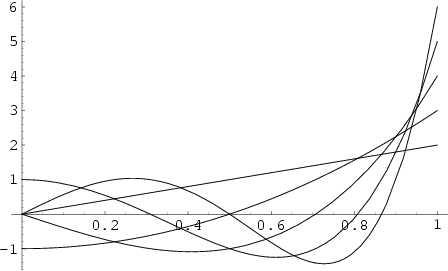A modified set of Chebyshev Polynomials defined by a slightly different Generating Function. Used to develop four-dimensional Spherical Harmonics in angular momentumtheory. They are also a special case of the Ultraspherical Polynomial with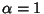. The Chebyshev polynomials of the second kind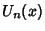are illustrated above for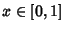and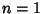, 2, ..., 5.

The defining Generating Function of the Chebyshev polynomials of the second kind is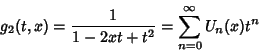(1)

for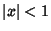and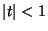. To see the relationship to a Chebyshev Polynomial of the First Kind (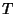), take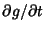,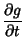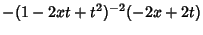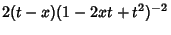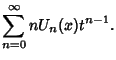(2)

Multiply (2) by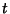,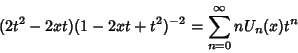(3)

and take (3) minus (2),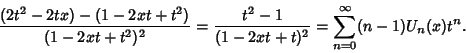(4)

The Rodrigues representation is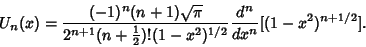(5)

The polynomials can also be written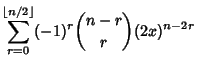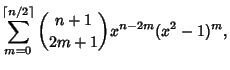(6)

where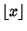is the Floor Function and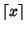is the Ceiling Function, or in terms of a Determinant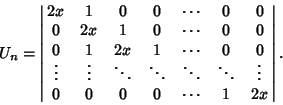(7)

The first few Polynomials are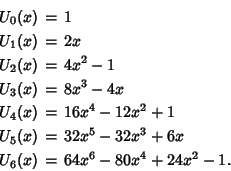Letting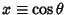allows the Chebyshev polynomials of the second kind to be written as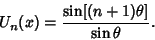(8)

The second linearly dependent solution to the transformed differential equation is then given by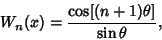(9)

which can also be written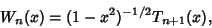(10)

where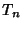is a Chebyshev Polynomial of the First Kind. Note that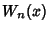is therefore not a Polynomial.

See also Chebyshev Approximation Formula, Chebyshev Polynomial of the First Kind, Ultraspherical Polynomial

References

Abramowitz, M. and Stegun, C. A. (Eds.). Orthogonal Polynomials.'' Ch. 22 in Handbook of Mathematical Functions with Formulas, Graphs, and Mathematical Tables, 9th printing. New York: Dover, pp. 771-802, 1972.

Arfken, G. Chebyshev (Tschebyscheff) Polynomials'' and Chebyshev Polynomials--Numerical Applications.'' §13.3 and 13.4 in Mathematical Methods for Physicists, 3rd ed. Orlando, FL: Academic Press, pp. 731-748, 1985.

Rivlin, T. J. Chebyshev Polynomials. New York: Wiley, 1990.

Spanier, J. and Oldham, K. B. The Chebyshev Polynomials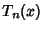and.'' Ch. 22 in An Atlas of Functions. Washington, DC: Hemisphere, pp. 193-207, 1987.